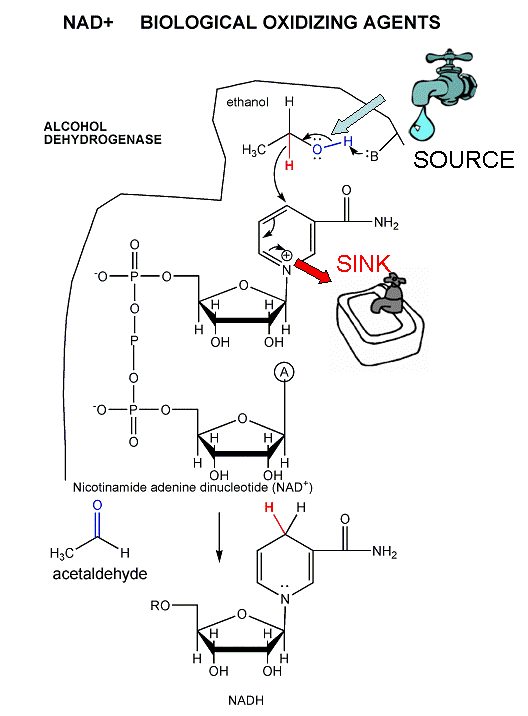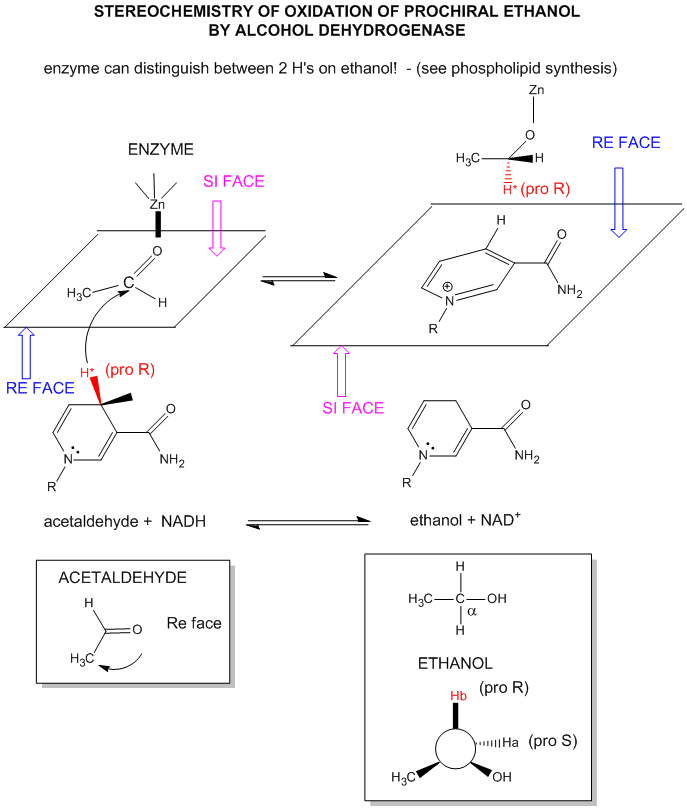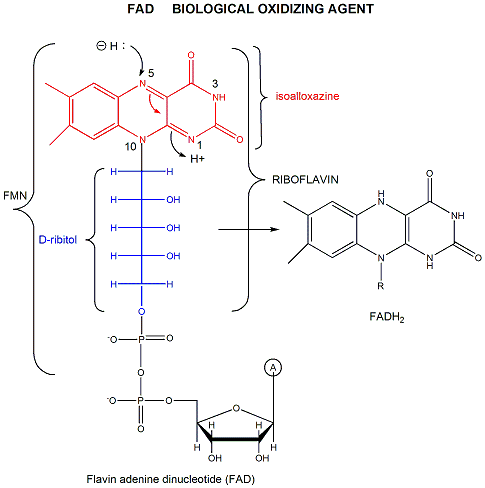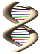# B3. Dehydrogenases

$$\newcommand{\vecs}{\overset { \rightharpoonup} {\mathbf{#1}} }$$ $$\newcommand{\vecd}{\overset{-\!-\!\rightharpoonup}{\vphantom{a}\smash {#1}}}$$$$\newcommand{\id}{\mathrm{id}}$$ $$\newcommand{\Span}{\mathrm{span}}$$ $$\newcommand{\kernel}{\mathrm{null}\,}$$ $$\newcommand{\range}{\mathrm{range}\,}$$ $$\newcommand{\RealPart}{\mathrm{Re}}$$ $$\newcommand{\ImaginaryPart}{\mathrm{Im}}$$ $$\newcommand{\Argument}{\mathrm{Arg}}$$ $$\newcommand{\norm}{\| #1 \|}$$ $$\newcommand{\inner}{\langle #1, #2 \rangle}$$ $$\newcommand{\Span}{\mathrm{span}}$$ $$\newcommand{\id}{\mathrm{id}}$$ $$\newcommand{\Span}{\mathrm{span}}$$ $$\newcommand{\kernel}{\mathrm{null}\,}$$ $$\newcommand{\range}{\mathrm{range}\,}$$ $$\newcommand{\RealPart}{\mathrm{Re}}$$ $$\newcommand{\ImaginaryPart}{\mathrm{Im}}$$ $$\newcommand{\Argument}{\mathrm{Arg}}$$ $$\newcommand{\norm}{\| #1 \|}$$ $$\newcommand{\inner}{\langle #1, #2 \rangle}$$ $$\newcommand{\Span}{\mathrm{span}}$$

Dehydrogenases enzymes usually involve NAD+/NADH and are named for the substrate that is oxidized by NAD+. For instance in the reaction:

$\text{pyruvate} + \text{NADH} \rightleftharpoons \text{lactate} + \text{NAD}^+$

which is used to regenerate NAD+ under anerobic conditions, the enzyme is named lactate dehydrogenase. As in acid/base reactions, when the preferred direction for the reaction (from a ΔGo perspective) is from stronger acid to weaker (conjugate) acid, the preferred direction for a redox reaction is in the direction from strong to weak oxidizing/reducing agents. This can easily be determined from charts of standard reduction potentials, and using the equation:

$ΔG^o = -nF ΔE^o$

• where $$F$$ is the Faraday constant (96,494 Coulombs/mol e- = 96, 494 J/(V.mol) = 23.06 kcal/(V.mol). One Faraday is the charge per one mol of electrons).
• and $$ΔE^o$$, the standard EMF or standard cell potential (total voltage at standard state conditions), which can be determined by adding the standard reduction potentials (Eo) for the two appropriate half-reactions, after reversing the equation for the half-reaction that represents the oxidation.

When n=2 (number of electrons) which is common for oxidations of organic molecules,

$ΔG^o (kcal/mol) = - 46.12\,ΔE^o$

or for government work

$ΔGo (kcal/mol) \approx - 50ΔE^o$

Notice when ΔEo > 0, ΔGo < 0, the reaction as written is favored under standard conditions. Note in the table below that many of the half reactions involve protons. For biological reactions involving free protons, the standard state concentration for the protons are not 1 M as for other solutes in solution, but defined to be the hydronium ion concentration at pH 7.0. The ΔEo and ΔGo values for the reactions involving hydrogen ions at a standard state of pH 7.0 are usually written as ΔEo' and ΔGo'

Table: Standard Reduction Potential Table (E0'), 25oC
oxidant

reductant

n (electrons) Eoï¿½ (volts)
Acetate + carbon dioxide

pyruvate

2 -0.70
succinate + CO2 + 2H+

α−ketoglutarate + H2O

2 -0.67
acetate

acetaldehyde

2 -0.60
glycerate-3-P glyceraldehyde-3-P + H2O 2 -0.55
O2 O2- 1 -0.45
ferredoxin (ox) ferredoxin (red) 1 -0.43
Carbon dioxide

formate

2 -0.42
2H+

H2

2 -0.42
α-ketoglutarate + CO2 + 2H+

isocitrate

2 -0.38
acetoacetate β-hydroxybutyrate 2 -0.35
Cystine

cysteine

2 -0.34
Pyruvate + CO2

malate

2 -0.33

2 -0.32

2 -0.32
FMN (enzyme bound) FMNH2 2 -0.30
Lipoic acid, ox

Lipoic acid, red

2 -0.29
1,3 bisphosphoglycerate + 2H+

glyceraldehyde-3-P + Pi

2 -0.29
Glutathione, ox

red

2 -0.23

2 -0.22
Acetaldehyde + 2H+

ethanol

2 -0.20
Pyruvate + 2H+

lactate

2 -0.19
Oxalacetate + 2H+

malate

2 -0.17
α-ketoglutarate + NH4+

glutamate

2 -0.14

2 0.003-0.09
Methylene blue, ox

Methylene blue, red

2 0.01
Fumarate + 2H+

succinate

2 0.03
CoQ (Ubiquinone - UQ + H+ UQH. 1 0.031
UQ + 2H+ UQH2 2 0.06
Dehydroascorbic acid

ascorbic acid

2 0.06
Ubiquinone; ox

red

2 0.10
Cytochrome b2; Fe3+

Cytochrome b2; Fe2+

1 0.12
Cytochrome c1; Fe3+

Cytochrome c1; Fe2+

1 0.22
Cytochrome c; Fe3+

Cytochrome c; Fe2+

1 0.25
Cytochrome a; Fe3+

Cytochrome a; Fe2+

1 0.29
1/2 O2 + H2O

H2O2

2 0.30
Cytochrome a3; Fe3+

Cytochrome a3; Fe2+

1 0.35
Ferricyanide

ferrocyanide

2 0.36
Cytochrome f; Fe3+

Cytochrome f; Fe2+

1 0.37
Nitrate

nitrite

1 0.42
Photosystem P700 . . 0.43
Fe3+

Fe2+

1 0.77
1/2 O2 + 2H+

H2O

2 0.816

The mechanism for the oxidation of a substrate by NAD + involves concerted hydride transfer to one face of NAD+.

Consider for example the oxidation of ethanol to acetaldehyde by alcohol dehydrogenase.Figure: oxidation of ethanol to acetaldehyde by alcohol dehydrogenase

For substrates like ethanol that loses a hydride from a methylene carbon atom that has two H's, only one of the H's is lost (either the proR or proS) from the prochiral center. (Remember the reaction of prochiral glycerol to give phospholipids.)FAD has a more positive reduction potential than NAD+ so it is used for more "demanding" oxidation reactions, such as dehydrogenation of a C-C bond to form an alkene. YouJmol: Updated Flavin dehydrogenase Jmol14 (Java) | JSMol (HTML5)